Reach Us+44-1474-556909
On Hydromagnetic Channel Flow of an Oldroyd-B Fluid Induced by Tooth Pulses in a Rotating System | OMICS International
Journal of Applied & Computational Mathematics
All submissions of the EM system will be redirected to Online Manuscript Submission System. Authors are requested to submit articles directly to Online Manuscript Submission System of respective journal.

# On Hydromagnetic Channel Flow of an Oldroyd-B Fluid Induced by Tooth Pulses in a Rotating System

Ghosh S*

Department of Computer Science, BIT Mesra, Kolkata Extension Centre, 1582 Rajdanga Main Road, Kolkata-700107, India

*Corresponding Author:
Ghosh S
Department of Computer Science
BIT Mesra, Kolkata Extension Centre
1582 Rajdanga Main Road, Kolkata-700107, India
Tel: 09885390953
E-mail: [email protected]

Received Date: March 13, 2015; Accepted Date: March 20, 2015; Published Date: April 20, 2015

Citation: Ghosh S (2015) On Hydromagnetic Channel Flow of an Oldroyd-B Fluid Induced by Tooth Pulses in a Rotating System. J Appl Computat Math 4:211. doi: 10.4172/2168-9679.1000211

Copyright: ©2015 Ghosh S. This is an open-access article distributed under the terms of the Creative Commons Attribution License, which permits unrestricted use, distribution, and reproduction in any medium, provided the original author and source are credited.

Visit for more related articles at Journal of Applied & Computational Mathematics

#### Abstract

An initial value problem concerning the motion of an incompressible electrically conducting viscoelastic Oldroyd-B fluid in a channel bounded by two infinite rigid non-conducting plates in presence of an external transverse magnetic field when both the fluid and the plates are in a state of solid body rotation with constant angular velocity about an axis normal to the plates is solved. The unsteady motion is generated impulsively from rest in such a fluid when the upper plate is subjected to velocity tooth pulses with the lower plate held fixed. It is assumed that no electric current exists in the basic state and the magnetic Reynolds number is very small. Exact solutions of the problem are obtained by utilizing two methods, of them, one is the method of Fourier analysis and the other is the method of Laplace transforms. The enquiries are made about the velocity field and the skin-friction on the walls. It is shown that both the methods give the same exact solution of the problem. The influence of rotation, the magnetic field and the elasticity of the fluid on the components of fluid velocity and the wall skin-frictions are examined quantitatively. Some known results are found to emerge as special cases of the present analysis.

#### Keywords

Hydromagnetic; Pulsatile flow; Oldroyd-B fluid; Rotating system

#### Introduction

The fluid flow generated by pulsatile motion of the boundary is found to have immense importance in aerospace science, nuclear fusion, astrophysics, geophysics and cosmical gas dynamics. The investigation in this direction was carried out by Chakraborty and Ray  who examined the magneto-hydrodynamic Couette flow of a viscous fluid between two parallel plates when one of the plates is set in motion by random pulses. Makar  presented the solution of magneto-hydrodynamic viscous flow between two parallel plates when one of the plates is subjected to velocity tooth pulses and the induced magnetic field is neglected. Bestman and Njoku  constructed the solution of hydromagnetic channel flow of an incompressible, electrically conducting viscous fluid produced by tooth pulses including the effect of induced magnetic field, ignored by the author , and using the methodology of Fourier analysis instead of applying the commonly used technique of Laplace transforms which involve complicated inversions. Ghosh and Debnath  considered the hydromagnetic channel flow of a two- phase fluid-particle system induced by tooth pulses and obtained solution using the method of Laplace transforms. Datta and Dalal [5,6] discussed the heat transfer to pulsatile flow of a dusty fluid in pipes and channels following the method of perturbation. On the other hand, Hayat et al.  studied some simple flows of an Oldroyd-B fluid using the method of Fourier transforms. Asgar et al.  also utilized the same methodology as that of authors  to solve the problem concerning Hall effects on the unsteady hydromagnetic flow of an Oldroyd-B fluid while Hayat et al.  constructed the solution of hydromagnetic Couette flow of an Oldroyd-B fluid in a rotating system following the method of perturbation. In the present paper, we intend to construct solution of hydromagnetic channel flow of a rotating Oldroyd-B fluid induced by tooth pulses with a view to its application in hydromagnetic spin-up in a contained fluid , the motion of the earth’s liquid core , the development of sunspot, the solar cycle and the structure of the magnetic stars  and in the determination of the effects of the external magnetic field and rotation on the flow of blood in the cardiovascular system, particularly at low rates of shear .

#### Basic Equations

Following Oldroyd  the constitutive equations for an oldroyd-B fluid can be written as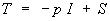(2.1)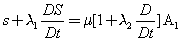(2.2)

Where T=Cauchy stress tensor, p=fluid pressure, I=identity tensor, S=extra stress tensor,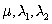= viscosity coefficient, relaxation time, retardation time (assumed constants).

The tensor A1 is defined as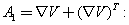(2.3)

In a cartesian system,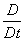(upper convected time derivative) operating on any tensor B1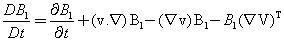(2.4)

It is to be mentioned here that this model includes the viscous fluid as a particular case for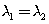; the Maxwell fluid when l2=0 and an oldroyd-B fluid when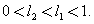The stress equations of motion for an incompressible electrically conducting oldroyd-B fluid in a rotating system in presence of an external magnetic fluid are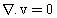(2.5)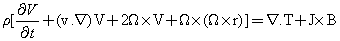(2.6)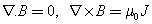(2.7ab)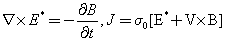(2.8ab)

where V=(u,v,w)=fluid velocity,=fluid density, J=current density, B=magnetic flux density, E*=electric field,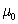=magnetic permeability (assumed constant), s0=electrical conductivity (assumed finite) and Ω=angular velocity of solid body rotation.

#### Formulation of the Problem

In view of the physical nature of the problem, as shown in Figure 1, we take

V=(u(z; t); v(z; t); 0); S=S(z; t); (3.1a)

B=(0; 0; B0); Ω=(0; 0; Ω) (3.1b)

Where z-axis is normal to the plate, x-axis is along the plate and y-axis perpendicular to it with origin at the plate.

The equation (2.2) then yields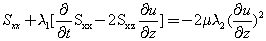(3.2a)(3.2b)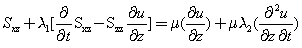(3.2c)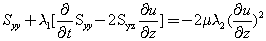(3.2d)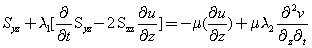(3.2e)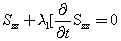(3.2f)

Integrating (3.2f), we get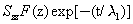(3.3)

Where F(z) is an arbitrary function of z. We shall investigate the possibility of the solution in which F(z)=0 i.e., Szz=0 .

We now assume that no applied or polarization voltage exists i.e, E*=0 so that no energy is added or extracted from the fluid by the electric field. We further assume that the magnetic Reynolds number is very small which is plausible for most electrically conducting fluids. This implies that the current is mainly due to induced electric field so that j=σ0(u×B) and the applied magnetic field remains essentially unaltered by the electric current flowing in the fluid. We also assume that the induced magnetic field produced by the motion of the fluid is negligible compared to the applied magnetic field so that the Lorentz force term in (2.6) becomes −σ 0 B02 V:

The equations of motion in (2.6) then reduces to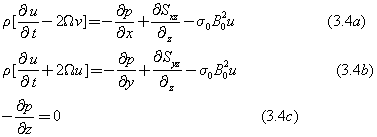Where p is the modified pressure including the centrifugal force term. The Equation (3.4c) shows that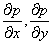have the same value as in the free stream. Accordingly, we assume that both the quantities are zero.

We now combine (3.4a) and (3.4b) with the help of (3.2c) and (3.2e) to obtain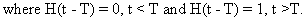(3.5)

Where q=u + i v is the complex velocity of the fluid.

We now introduce the non-dimensional quantities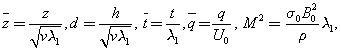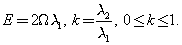in equation (3.5) and on dropping bars we get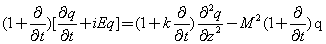(3.6)

The problem now reduces to solving (3.6) subject to the boundary and initial conditions given by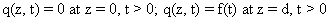(3.7 a,b)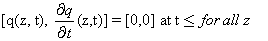(3.7c)

where f(t) representing the tooth pulses is an even periodic function with period 2T and strength E0 T.

#### Solution of the Problem

Method of fourier analysis

According to the nature of f(t) mentioned above, the mathematical form of u(d, t) may be written as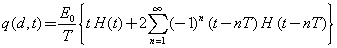(4.1)Using half-range Fourier series, the condition (4.1) can also be expressed in the form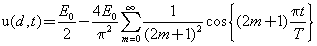(4.2)

By virtue of (4.2) we assume the solution of (3.6) as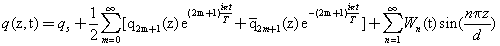(4.3)

Where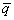is the conjugate of q. The first two terms in (4.3) are chosen so as to satisfy (4.2)

While the last term accommodates the initial condition.

Substituting (4.3) in (3.6) and then using (3.7a) and (4.2), we have the following equations

With appropriate conditions as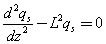(4.4)

With qs=0 on z=0;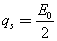on z=d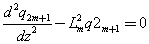(4.5)

With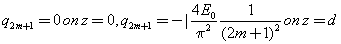And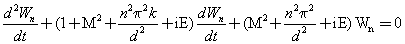(4.6)

With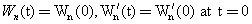Where Wn(0) and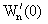are to be determined.

In the above,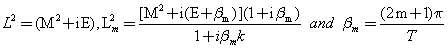The solutions of equations (4.4)-(4.6) are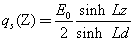(4.7)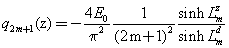(4.8)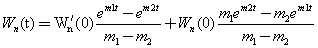(4.9)

Where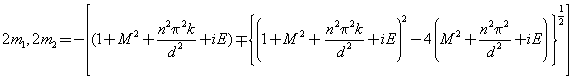(4.10)

The initial conditions in (3.7b) provide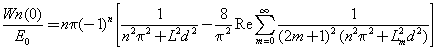(4.11)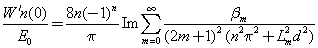(4.12)

The m-series in (4.11) and (4.12) are of orders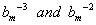when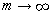. The n-series is also convergent since m1; m2 are of order -N12 and m1-m2 has the order N1 2 as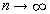where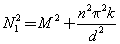Finally, the fluid velocity takes the form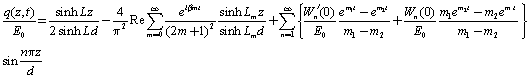(4.13)

which in the limit t → ∞ provides the steady velocity field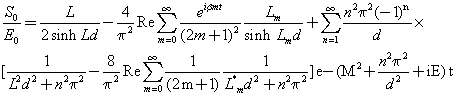(4.14)

where the harmonic part contains the effect of fluid elasticity due to the presence of pulsation.

On the other hand, the solution corresponding to classical viscous fluid in a rotating system can be obtained from (4.13) in the limit k → 1. This solution is given by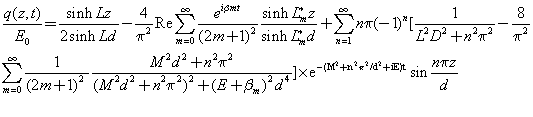(4.15)

Where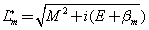The result (4.15) in a non-rotating system (E=0) yields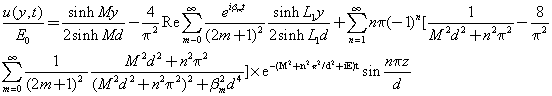(4.16)

Where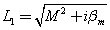The solution (4.16) is identical to that of Bestman and Njoku . In particular, when T → 0 and E0=2 the general result (4.13) reduces to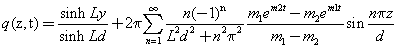(4.17)

which describes the hydromagnetic channel flow of a rotating Oldroyd-B fluid generated by impulsive motion of the upper plate with a constant velocity. The result (4.17) in dimensionless form and in the limit M → 0; E → 0 coincides exactly with that of authors  while the result (4.16) in the limit T → 0 and E0=2V agrees completely with that of Soundalgekar .

The skin-friction on the plates z=0 and z=d are given by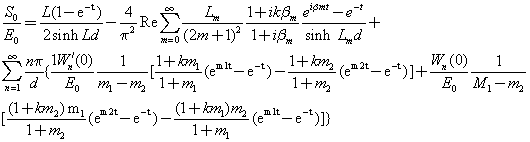(4.18)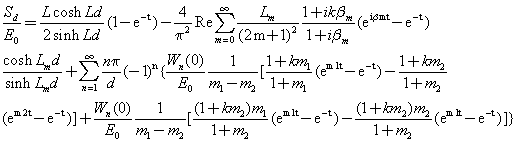(4.19)

Above results in the limit k → 1 (viscous fluid) reduces to(4.20)

And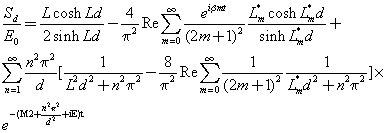(4.21)

However, when T→0 and E0=2, (4.20) and (4.21) provide the hydromagnetic solutions in a rotating system which are given by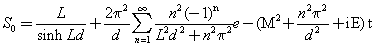(4.22)

And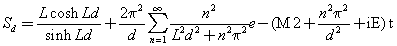(4.23)

Finally, when E → 0, (4.22) and (4.23) provide the classical results as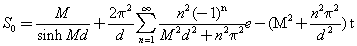And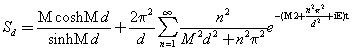(4.24)

II. Method of Laplace transforms

The problem, when solved by the method of Laplace transform technique, reduces to solving the transformed equation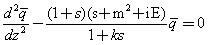(4.25)

subject to the conditions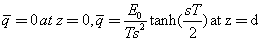(4.26)

The expression for the fluid velocity q(z,t) then obtained as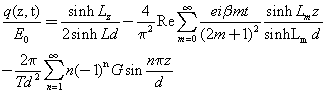(4.27)

Where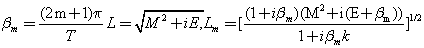G=G1 + G2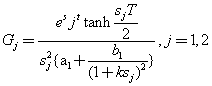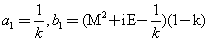However, in the limit k → 1,the result (4.27) reduces to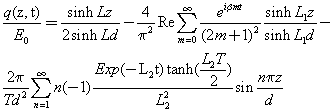(4.28)

Where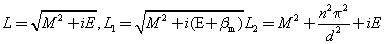The result (4.28) is in excellent agreement with those of author  and authors  in the limit E → 0.

The corresponding expressions for skin-friction at the plates are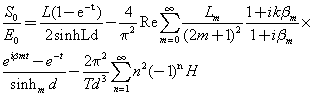(4.29)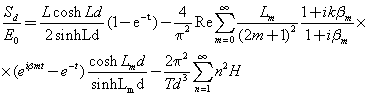(4.30)

where L=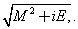,H=H1 + H2;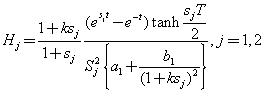(4.31)

The result (4.13) obtained by the method of Fourier analysis when compared with (4.27) obtained by the method of Laplace transforms reveals that the two results are exactly identical in respect of their steady and harmonic parts but the transient parts of them are of different forms. In order to show that these two results for the fluid velocity represent the same exact solution of the problem we incorporate the analysis given in the appendix.

#### Numerical Results and Discussions

The nature of pulses subjected on the upper plate produces both the developing (increasing) and the retarding (decreasing) flows in the fluid. To investigate the effects of various flow parameters on the fluid velocity corresponding to developing flow at t=0.5 and the retarding flow at t=1.75, the exact solution (4.13) is evaluated for the cases E=0, E=0.1 and E=1.0 when T=1. The non-zero values of flow parameters are chosen arbitrarily within their range of validity. The changing nature of the velocity components are incorporated in Figures 2 and 4(a,b) to 6(a,b) for different values of the fluid elasticity k, the magnetic field M and the rotation E.

Figure 3: Fluid velocity component u/E0for different values of (t, M, k) in a non-rotating system (E=0) when T=1.0, d=1.

Figure 4a: Fluid velocity component u/E0for different values of (t, M, k) in a rotating system (E=0.1) when T =1.0, d=1.

Figure 4b: Fluid velocity component u/E0for different values of (t, M, k) in a rotating system (E=0.1) when T =1.0, d=1.

Figure 5a: Fluid velocity component u/E0for different values of (t, M, k) in a rotating system (E=1.0) when T =1.0, d=1.

Figure 5b: Fluid velocity component u/E0for different values of (t, M, k) in a rotating system (E=1.0) when T =1.0, d=1.

Figure 6b: Fluid velocity component u/E0for different values of (E,t) when T =1.0, M= 0.5, k= 0.4, d=1.

It is observed from Figure 3 that in absence of rotation (E=0) and for fixed values of the magnetic field M, the decrease in elastic parameter k decreases the fluid velocity u=E0 when the flow is developing and increases the same when the flow is retarding. Such a result is expected because the fluid is more and more viscoelastic for smaller and smaller values of k with k=1 representing the clean viscous fluid and the viscoelastic fluids neither grow nor decay as quickly as clean viscous fluids. It is further noticed that the effect of k on u=E0 whether decreasing or increasing enhances with the increase of the magnetic field although the magnetic field has an overall damping effect on the flow for fixed values of k. As a result the effect of k persists even when the steady state is attained (eqn.(4.14)).

However, in presence of rotation, the fluid velocity component u=E0 varies in a manner similar to that of non-rotating case excepting a significant diminution in its magnitude with the increase of rotation for all values of k and M when the flow is developing. On the contrary, exactly reverse effect is found when the flow is retarding. On the other hand, the increase in magnetic field continues to produce its damping effect on all kinds of flow in presence of rotation and the elasticity k of the fluid. The above findings are exhibited in Figures 4a and 5a. More specifically, for fixed values of M,k,T, the decreasing and increasing effects of rotation E on the component of fluid velocity u=E0 respectively for the increasing and decreasing motion is shown in Figure 6a.

It is noticed that the lateral component of fluid velocity v=E0 appears in a contained fluid only in presence of rotation. In the developing flow, the magnitude of v=E0 increases with the increase of rotation E and the elasticity k but decreases with the increase of the magnetic field M. A reverse effect with respect to elastic parameter k is found when the flow is retarding. The variation of fluid velocity component v=E0 for different values of flow parameters and time is illustrated in Figures 4b and 5b while the increasing effects of rotation E for fixed values of (M,k,T) on the magnitude of v=E0 both for the increasing and decreasing motions are presented in Figure 6b.

To investigate the effects of various flow parameters on the components of skin-friction on the plates, the results (4.18) and (4.19) are evaluated numerically for the case E=1.0 when T=1.0 and d=1. The results are presented in Figures 7a to 10b. It is observed from Figures 7a and 8a that the longitudinal component of skin-friction on both the plates fluctuate in a manner similar to that of pulses imparted in the fluid. On the lower plate, the longitudinal component of skinfriction decreases with the decrease of the elasticity k for all values of the magnetic field M when the flow is developing but a reverse effect is found when the flow is retarding. On the contrary, the magnitude of the longitudinal component of the skin-friction at the upper plate although decreases with decrease of the elasticity k but increases with the increase of the magnetic field M when the flow is developing and a reverse effect with respect to the elasticity k is found for all values of the magnetic field M when the flow is retarding. The curves corresponding to M=0.0 and k=1.0 in Figures 7a and 8a represent the quantitative response of the longitudinal component of skin-friction respectively on the lower and the upper plate for the case of classical viscous fluid.

Figure 7a: Longitudinal component of skin friction S0/E0at the lower plate for different values of (M, k) when T =1.0, d=1.

Figure 7b: Lateral component of skin friction S0/E0at the lower plate for different values of (M, k) when T =1.0, d=1.

Figure 8a: Longitudinal component of skin friction Sd/E0at the upper plate for different values of (M, k) when T =1.0, d=1.

Figure 8b: Lateral component of skin friction Sd/E0 at the upper plate for different values of (M, k) when T =1.0, d=1.

Figure 9a: Variation of Longitudinal component of skin friction S0/E0 at the lower plate with rotation (E) for fixed values of (T, M, k) and d=1.

Figure 9b: Variation of Lateral component of skin friction S0/E0at the lower plate with rotation (E) for fixed values of (T, M, k) and d=1.

Figure 10a: Variation of Longitudinal component of skin friction Sd/E0at the upper plate with rotation (E) for fixed values of (T, M, k) and d=1.

Figure 10b: Variation of Lateral component of skin friction Sd/E0at the upper plate with rotation (E) for fixed values of (T, M, k) and d=1.

It is further noticed from Figure 9a that for fixed values of M,k,T, the longitudinal components of the skin-friction at the lower plate decrease with the increase of rotation E when the flow is developing but increase with E when the flow is retarding. However, a reverse effect is found at the upper plate which is evident from Figure 10a. On the other hand, the magnitude of lateral components of skin-frictions on both the plates increase with the increase of the elastic parameter k for all values of the magnetic field M as evidenced from Figures 7b and 8b. However, the lateral component of skin-frictions are negative at the lower plate and positive at the upper plate for all values of M and k. Finally, for fixed values of (M,k,T) the magnitude of the lateral component skin-frictions on both the plates increases with the increase of rotation E as exhibited in Figures 9b and 10b.

#### Conclusion

An analysis concerning the hydromagnetic channel flow of an Olroyd-B fluid induced by tooth pulses in a rotating system has been presented in this paper. Both the method of Fourier analysis and the method of Laplace transforms are adopted to arrive at the solution of the problem separately. It is shown that both the methods provide the same exact solution of the problem. The quantitative analysis of the results are incorporated following the solutions obtained by the method of Fourier analysis not due to compulsion. One can also use the solutions obtained by the method of Laplace transforms to explain the results quantitatively. The present analysis seems to be useful in various branches of science and technology with particular reference to geophysical and physiological fluid flow situations.

#### References

Select your language of interest to view the total content in your interested language

### Article Usage

• Total views: 12101
• [From(publication date):
April-2015 - Sep 20, 2019]
• Breakdown by view type
• HTML page views : 8298

## Post your commentCan't read the image? click here to refresh
###### Peer Reviewed Journals

Make the best use of Scientific Research and information from our 700 + peer reviewed, Open Access Journals

International Conferences 2019-20

Meet Inspiring Speakers and Experts at our 3000+ Global Annual Meetings

Top